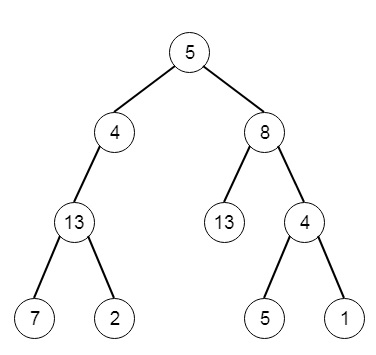# Path Sum III in C++

Suppose we have given a binary tree in which each node holds an integer key. We have to find the paths that sum to a given value. The path should start from root to leaf. We have to find the path where the sum is same.

If the tree is like [5,4,8,11,null,13,4,7,2,null,null,5,1], and sum is 22, then it will be −The paths are [[5,4,11,2],[5,8,4,5]].

To solve this, we will follow these steps −

• Use the dfs function to solve this problem, the dfs is slightly modified, this will work as follows. This function will take the root, sum, and one temp array
• if root is not present, then return
• if left of root and right of root are empty, then
• if sum = value of root, then
• insert value of root into temp, insert temp into result, and delete the last node from the temp
• return
• insert value of root into the temp
• dfs(left of root, sum – value of root, temp)
• dfs(right of root, sum – value of root, temp)
• delete the last element from temp

Let us see the following implementation to get better understanding −

## Example

Live Demo

#include <bits/stdc++.h>
using namespace std;
void print_vector(vector<vector<int> > v){
cout << "[";
for(int i = 0; i<v.size(); i++){
cout << "[";
for(int j = 0; j <v[i].size(); j++){
cout << v[i][j] << ", ";
}
cout << "],";
}
cout << "]"<<endl;
}
class TreeNode{
public:
int val;
TreeNode *left, *right;
TreeNode(int data){
val = data;
left = right = NULL;
}
};
void insert(TreeNode **root, int val){
queue<TreeNode*> q;
q.push(*root);
while(q.size()){
TreeNode *temp = q.front();
q.pop();
if(!temp->left){
if(val != NULL)
temp->left = new TreeNode(val);
else
temp->left = new TreeNode(0);
return;
} else {
q.push(temp->left);
}
if(!temp->right){
if(val != NULL)
temp->right = new TreeNode(val);
else
temp->right = new TreeNode(0);
return;
} else {
q.push(temp->right);
}
}
}
TreeNode *make_tree(vector<int> v){
TreeNode *root = new TreeNode(v);
for(int i = 1; i<v.size(); i++){
insert(&root, v[i]);
}
return root;
}
class Solution {
public:
vector < vector <int> > res;
void dfs(TreeNode* root, int sum, vector <int>& temp){
if(!root)return;
if(!root->left && !root->right){
if(sum == root->val){
temp.push_back(root->val);
res.push_back(temp);
temp.pop_back();
}
return;
}
temp.push_back(root->val);
dfs(root->left, sum - root->val, temp);
dfs(root->right, sum - root->val, temp);
temp.pop_back();
}
vector<vector<int>> pathSum(TreeNode* root, int sum) {
res.clear();
vector <int> temp;
dfs(root, sum, temp);
return res;
}
};
main(){
Solution ob;
vector<int> v = {5,4,8,11,NULL,13,4,7,2,NULL,NULL,NULL,NULL,5,1};
TreeNode *root = make_tree(v);
print_vector(ob.pathSum(root, 22));
}

## Input

[5,4,8,11,null,13,4,7,2,null,null,5,1]
22

## Output

[[5, 4, 11, 2, ],[5, 8, 4, 5, ],]

Updated on: 04-May-2020

130 Views